• 条件熵
千次阅读
2021-11-27 17:57:10

## 信息熵

信息熵，可以用来衡量一个信息的复杂程度，通俗的理解就是表示了这件事的不确定性，如果其不确定性越大，则信息熵的值也就越大，反之，则越小。
对于随机事件X，用 p x p_{x} 表示事件x发生的概率，则随机事件X的信息熵可以表示为：
H ( X ) = − ∑ i = 0 n p x l o g p x {\Large H(X) = - \sum_{i=0}^{n}p_{x} logp_{x}}
用python实现信息熵：

#计算信息熵,data为series对象
def getEntropy(data):
if not isinstance(data,pd.core.series.Series):
s = pd.Series(data)
prt_ary = data.groupby(data).count().values / float(len(data))
return sum(-(np.log2(prt_ary)*prt_ary))


## 条件熵

条件熵，表示在已知随机变量X的情况下，随机变量Y的不确定性。公式定义如下：
H ( Y ∣ X ) = ∑ x p x H ( Y ∣ X = x ) {\Large H(Y|X)=\sum_{x}^{} p_{x} H(Y|X=x)}
python 实现条件熵的代码如下：

def getCondEntropy(data,xname,yname):
xs = data[xname].unique()
ys = data[yname].unique()
p_x = data[xname].value_counts() / data.shape
ce = 0

for x in xs:
ce += p_x[x]*getEntropy(data[data[xname] == x][yname])
print(str(x) + ":" + str(p_x[x]*getEntropy(data[data[xname] == x][yname])))

return cepython 机器学习 条件熵
更多相关内容
• ConditionalEntropy：给定 X，计算 Y 的条件熵（以位为单位） H = 条件熵（Y，X） H = Y 的计算熵，给定 X（以位为单位） Y = 因变量（列向量） X = 自变量注 1：每个不同的值都被视为一个唯一的符号。 注 2：需要...matlab
• matlab代码，包含功能：计算互信息、香农熵、Renyi熵、条件熵、加权熵、加权互信息等。用法举例： >> y = [1 1 1 0 0]'; >> x = [1 0 1 1 0]'; >> mi(x,y) %% mutual information I(X;Y) >> h(x) %% entropy H(X) >>...
• 函数 eCE = CondEn( x, delay, order, windowSize ) 从滑动窗口中的 1D 时间序列有效地计算序数模式的条件熵，用于顺序模式的 1...8 阶 。 在...matlab
• 通过分析信息熵在异常检测中的应用和条件熵的固有特性，提出了一种基于条件熵和加权熵理论的异常检测方法。分析网络流量特征，选取具有内在相关性的网络特征属性，给出了加权系数矩阵的公式和异常检测的具体实现方法...异常检测 流量分析
• 以分类为基础提出了一种基于条件熵的决策表属性并行约简算法。该算法通过条件熵的计算在属性约简的同时将原决策表逐层分解为相对于决策属性来说尽量均匀的子决策表，从而实现了属性约简的并行计算。随后对该算法的...决策表 属性约简 条件熵 并行计算
• ## 条件熵

千次阅读 2018-12-05 20:13:00
条件熵 1 信息熵以及引出条件熵 我们首先知道信息熵是考虑该随机变量的所有可能取值，即所有可能发生事件所带来的信息量的期望。公式如下： 我们的条件熵的定义是：定义为X给定条件下，Y的条件...

# 条件熵

## 1  信息熵以及引出条件熵

我们首先知道信息熵是考虑该随机变量的所有可能取值，即所有可能发生事件所带来的信息量的期望。公式如下：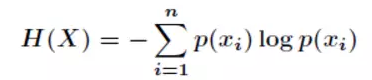我们的条件熵的定义是：定义为X给定条件下，Y的条件概率分布的熵对X的数学期望

这个还是比较抽象，下面我们解释一下：

设有随机变量（X,Y），其联合概率分布为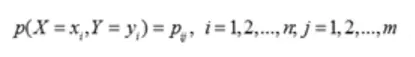条件熵H（Y|X）表示在已知随机变量X的条件下随机变量Y的不确定性。

随机变量X给定的条件下随机变量Y的条件熵H(Y|X)

通俗来讲就是，知道X情况下，Y的信息量。

## 2  公式

下面推导一下条件熵的公式：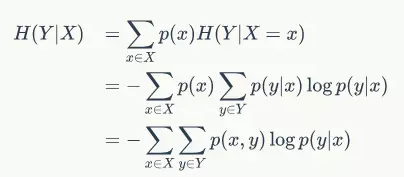## 3  注意

注意，这个条件熵，不是指在给定某个数（某个变量为某个值）的情况下，另一个变量的熵是多少，变量的不确定性是多少？而是期望！

因为条件熵中X也是一个变量，意思是在一个变量X的条件下（变量X的每个值都会取），另一个变量Y熵对X的期望。

这是最容易错的！

## 4  例子

下面通过例子来解释一下：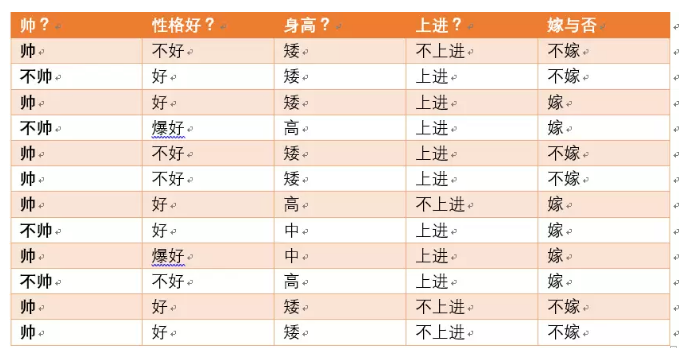假如我们有上面数据：

设随机变量Y={嫁，不嫁}

我们可以统计出，嫁的个数为6/12 = 1/2

不嫁的个数为6/12 = 1/2

那么Y的熵，根据熵的公式来算，可以得到H（Y） =  -1/2log1/2 -1/2log1/2

为了引出条件熵，我们现在还有一个变量X，代表长相是帅还是帅，当长相是不帅的时候，统计如下红色所示：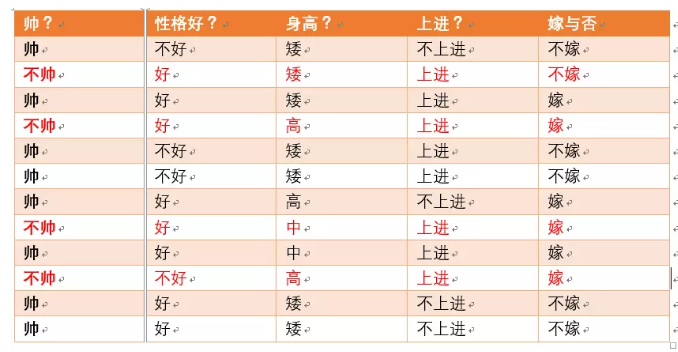可以得出，当已知不帅的条件下，满足条件的只有4个数据了，这四个数据中，不嫁的个数为1个，占1/4

嫁的个数为3个，占3/4

那么此时的H（Y|X = 不帅） = -1/4log1/4-3/4log3/4

p(X = 不帅) = 4/12 = 1/3

同理我们可以得到：

当已知帅的条件下，满足条件的有8个数据了，这八个数据中，不嫁的个数为5个，占5/8

嫁的个数为3个，占3/8

那么此时的H（Y|X = 帅） = -5/8log5/8-3/8log3/8

p(X = 帅) = 8/12 = 2/3

## 5  计算结果

有了上面的铺垫之后，我们终于可以计算我们的条件熵了，我们现在需要求：

H（Y|X = 长相）

也就是说，我们想要求出当已知长相的条件下的条件熵。

根据公式我们可以知道，长相可以取帅与不帅俩种

条件熵是另一个变量Y熵对X（条件）的期望。

公式为：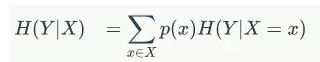H（Y|X=长相） = p(X =帅)*H（Y|X=帅）+p(X =不帅)*H（Y|X=不帅）

然后将上面已经求得的答案带入即可求出条件熵！

这里比较容易错误就是忽略了X也是可以取多个值，然后对其求期望！！

## 6  总结

其实条件熵意思是按一个新的变量的每个值对原变量进行分类，比如上面这个题把嫁与不嫁按帅，不帅分成了俩类。

然后在每一个小类里面，都计算一个小熵，然后每一个小熵乘以各个类别的概率，然后求和。

我们用另一个变量对原变量分类后，原变量的不确定性就会减小了，因为新增了Y的信息，可以感受一下。不确定程度减少了多少就是信息的增益。

原文 知乎专栏：https://zhuanlan.zhihu.com/p/26551798

posted @ 2018-12-05 20:13 小时候挺菜 阅读( ...) 评论( ...) 编辑 收藏
展开全文• 【机器学习】用相亲样本数据集详解条件熵的计算过程。 使用的样本集简介如下： 样本集简介： 样本集有8个example样本 每个样本有3个特征(身高，房子，性格)，1个分类结果refuse或者agree 身高取值范围={high, ...
• 《统计机器学习》第六章最大熵模型，对和有关的公式的解惑

## 1、问题描述

在读李航的《统计机器学习》第六章最大熵模型时看到一个矛盾的地方：
关于熵的描述：

H ( P ) = − ∑ i n P ( x i ) l o g P ( x i ) H(P)=-\sum_{i}^{n}P(x_i)logP(x_i)

而定义在条件概率分布 P ( Y ∣ X ) P(Y|X) 上的条件熵又写为：

H ( P ) = − ∑ x , y P ~ ( x ) P ( y ∣ x ) l o g P ( y ∣ x ) H(P)=-\sum_{x,y}\tilde{P}(x)P(y|x)logP(y|x)

但是按照我的理解应该写为：

H ( P ) = − ∑ x , y P ( y ∣ x ) l o g P ( y ∣ x ) H(P)=-\sum_{x,y}P(y|x)logP(y|x)

感觉书本上的公式多了个 P ~ ( x ) \tilde{P}(x) 项。查阅资料发现我还是太浅薄了哈哈 数学不行，没有真正理解 H ( P ) H(P) 的含义。

## 2、公式推导

条件熵 H ( Y ∣ X ) H(Y|X) 表示在已知随机变量 X X 的条件下随机变量 Y Y 的不确定性。条件熵 H ( Y ∣ X ) H(Y|X) 定义为 X X 给定条件下 Y Y 的条件概率分布的熵对 X X 的数学期望：

H ( Y ∣ X ) = ∑ x p ( x ) H ( Y ∣ X = x ) = − ∑ x p ( x ) ∑ y p ( y ∣ x ) l o g p ( y ∣ x ) = − ∑ x ∑ y p ( x ) p ( y ∣ x ) l o g p ( y ∣ x ) = − ∑ x , y p ( x ) p ( y ∣ x ) l o g p ( y ∣ x ) = − ∑ x , y p ( x , y ) l o g p ( y ∣ x ) H(Y|X)=\sum_{x}p(x)H(Y|X=x) \\=-\sum_{x}p(x)\sum_{y}p(y|x)logp(y|x) \\=-\sum_{x}\sum_{y}p(x)p(y|x)logp(y|x) \\=-\sum_{x,y}p(x)p(y|x)logp(y|x) \\=-\sum_{x,y}p(x,y)logp(y|x)

其实化简到倒数第二步已经和书本上一样了，最后一步是因为 p ( y ∣ x ) p ( x ) = p ( x , y ) p(y|x)p(x)=p(x,y) .
重要的是要理解 H ( Y ∣ X ) = ∑ x p ( x ) H ( Y ∣ X = x ) H(Y|X)=\sum_{x}p(x)H(Y|X=x)

展开全文机器学习 人工智能
• 关于条件熵的质疑，王勇，，从多个角度分析了条件熵的定义和计算公式的问题，对条件熵的计算公式进行了纠正，并且举例证明了熵不增并不是绝对的，进而指出香
• 离散型随机变量的熵：熵是一个随机变量不确定性的度量，一个随机变量的熵越大，...条件熵H(Y|X)表示在已知事件X的条件下，事件Y的不确定性，即X发生的前提下，Y发生的熵。随机变量X = [0.1;0.7;0.5;0.1]，随机变量Y =

# 1、单变量的熵

离散型随机变量的熵：

熵是一个随机变量不确定性的度量，一个随机变量的熵越大，意味着不确定性越大，换言之，该随机变量包含的信息量越大。必然事件是确定无疑的，并不含有不确定性，所以必然事件的熵应该是0，也就是说，必然事件不含有信息量。

# 2、联合熵

离散型的二维随机变量(X,Y)的联合熵为：

如果想要考虑两个随机变量组合一起的不确定性，得用到联合熵。

# 3、条件熵

并且：

因此我们可以得到：

条件熵H(Y|X)表示在已知事件X的条件下，事件Y的不确定性，即X发生的前提下，Y发生的熵。

# 4、实例讲解

随机变量X = [0.1;0.7;0.5;0.1]，随机变量Y = [2;32;6666;5]。

现在通过直方图方式计算单个向量的直方图分布：

假设X分为四个区间，X每个区间大小为(0.7 - 0.1) / 4 = 0.15，因此区间的分界值为[-inf;0.25;0.4;0.55;inf]，落入每个区间的样本数为[2;0;1;1]，每个样本落入各自区间的区间号为[1;4;3;1]，因此p(X) = [0.5;0;0.25;0.25]。

假设Y分为四个区间，Y每个区间大小为(6666 - 2) / 4 = 1666，因此区间的分界值为[-inf;1668;3334;5000;inf]，落入每个区间的样本数为[3;0;0;1]，每个样本落入各自区间的区间号为[1;1;4;1]，因此p(Y) = [0.75;0;0;0.25]。

根据X和Y每个样本落入的区间情况，落入(1,1)有两个样本，落入(4,1)有一个样本，落入(3,4)有一个样本：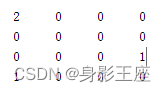X和Y的联合分布概率如下，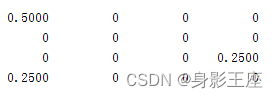所以：H(X|Y) = H(XY)-H(Y) = 1.5 - 0.8133 = 0.6867。IG(X|Y) = H(X) - H(X|Y) =1.5 - 0.6867 = 0.8133。SU = 2 * IG(X|Y) / (H(X) + H(Y)) = 2 * 0.8133 / (1.5 + 0.8133) = 0.703。

IG互信息描述了一个随机变量包含另一个的信息量。由于它可以很好地描述随机变量之间的非线性关系，因此该测度方法常被用来描述特征与类标签之间或特征之间的相关关系。然而，它对变量的规模很敏感。SU可以通过将互信息归一化来弥补上述缺点。目前，SU已成功应用于各种FS算法。

# 5、编程实现

function mi = calmi(u1, u2, wind_size)
x = [u1, u2];
n = wind_size;
[xrow, xcol] = size(x);
bin = zeros(xrow,xcol);
pmf = zeros(n, 2);
for i = 1:2
minx = min(x(:,i));
maxx = max(x(:,i));
binwidth = (maxx - minx) / n;
edges = minx + binwidth*(0:n);
histcEdges = [-Inf edges(2:end-1) Inf];
[occur,bin(:,i)] = histc(x(:,i),histcEdges,1); %通过直方图方式计算单个向量的直方图分布
pmf(:,i) = occur(1:n)./xrow;
end
%计算u1和u2的联合概率密度
jointOccur = accumarray(bin,1,[n,n]);  %（xi，yi）两个数据同时落入n*n等分方格中的数量即为联合概率密度
jointPmf = jointOccur./xrow;
Hx = -(pmf(:,1))'*log2(pmf(:,1)+eps);
Hy = -(pmf(:,2))'*log2(pmf(:,2)+eps);
Hxy = -(jointPmf(:))'*log2(jointPmf(:)+eps);
MI = Hx+Hy-Hxy;
%mi = MI/sqrt(Hx*Hy);% 标准化互信息，两种形式 NMI=2*MI/(Hx+Hy) 和 NMI=MI/sqrt(Hx*Hy)
mi = 2*MI/(Hx+Hy);
end
% function: main.m
clc;clear;clearvars;
X = [0.1;0.7;0.5;0.1];
Y = [2;32;6666;5];
% 划分的区间数
wind_size = size(X,1);
mi = calmi(X, Y, wind_size);
disp(mi);

输出：0.7020

由于计算时候，有四舍五入现象，所以有点出入，但是实现没有任何问题。至此求两个变量之间的标准互信息或者SU，就已经顺利完成了。

展开全文人工智能
• 具有定向传递函数和校正后的条件熵的皮层皮质和脑心功能耦合的估计
• 为了准确检测这种攻击的存在，提出了基于条件熵和 GHSOM（growing hierarchical SOM）神经网络的 DDoS 攻击检测方法MBCE&G 。首先，依据此DDoS的阶段性特征，定位了网络中的受损交换机以发现可疑攻击流；然后，依据...条件熵 神经网络 DDoS攻击
• MATLAB实现条件熵的线性估计函数，可用于非线性系统的条件熵估计MATLAB
• ## 信息熵和条件熵的计算

万次阅读 多人点赞 2019-07-12 19:34:58条件熵 机器学习
• 一、信息 先抛出信息公式如下： 其中P(xi)P(x_i)P(xi​)代表随机事件X为xix_ixi​的概率，下面来逐步介绍信息的公式来源！ 信息量 信息量是对信息的度量，就跟时间的度量是秒一样，当我们考虑一个离散的随机...
• 8.5.1复合 前面（8.2.2）式得出了仅有一个随机变量情况下的公式（8.2）。如果抽样实验是由两个（或者多个）实验复合而成（对应于两个集合的笛卡尔积），就存在一个联合概率分布。例如掷一枚硬币还掷一次骰子，...
• 1948年，香农在著名的论文“通信的数学原理”中首次提出了（Entropy）的概念，这也解决了信息的度量问题，并量化了信息的作用。 信息 一条信息的信息量的多少，在直观上我们认为是和内容的多少有关，科学一点讲...
• 计算两个离散变量x和y的条件熵z = H（x | y）。 with Input：两个相同长度的整数向量 有益于学习
• 转自：通俗理解条件熵 前面我们总结了信息熵的概念通俗理解信息熵 - 知乎专栏,这次我们来理解一下条件熵。 我们首先知道信息熵是考虑该随机变量的所有可能取值，即所有可能发生事件所带来的信息量的期望。公式如下...python 机器学习
• 大家或多或少都听过一些熵的概念和定义，但是可能对他们的关系不是很清楚，本文就熵，联合熵，条件熵，互信息的推导展开介绍。 熵 H(X)=−∑xεXP(x)log⁡P(x) H(X)=-\sum_{x\varepsilon X}{P}(x)\log P(x) H(X)=−x...机器学习
• 熵值计算的matlab 程序包括样本熵，近似熵，条件熵
•机器学习
• 决策树： 信息熵：表示的是一个随机变量可能取值的期望，期望越大表示一个系统越混乱，期望越小... 条件熵：给定条件X的情况下，所有不同x值情况下Y的信息熵的平均值叫做条件熵条件熵 = 联合熵 - 信息熵 信息增益决策树 机器学习
• 分析了增加新对象后,决策表的决策属性关于条件属性的条件熵变化原理.并在此基础上提出了一种新的增量核求解算法.该算法只需找到与新对象属性值相等的条件类和决策类就可以得到新的条件熵,进而求得决策表在信息观下的...
• 信息论条件熵、联合熵及熵的性质课件 信息论条件熵、联合熵及熵的性质课件 信息论条件熵、联合熵及熵的性质课件 信息论条件熵、联合熵及熵的性质课件
• 3、条件熵 定义：在一个条件下，随机变量的不确定性。 4、信息增益 在一个条件下，信息不确定性减少的程度 H（X,Y） = H（x）-H（Y|X） 5、互信息 定义：指的是两个随机变量之间的相关程度。 ...信息增益 决策树
• 多尺度采样计算程序,该程序结合采样程序可以计算在多尺度条件下的的结果...